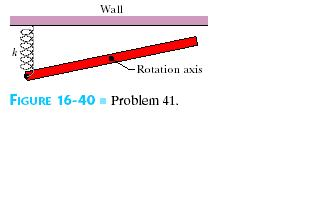# In the overhead view of, a long uniform rod of mass m0.6 Kg is free to rotate in a horizontal planeabout a vertical axis through its center .A springSinead Mcgee 2021-05-10 Answered
In the overhead view of, a long uniform rod of mass m0.6 Kg is free to rotate in a horizontal planeabout a vertical axis through its center .A spring with force constant k = 1850 N/m is connected horizontally betweenone end of the rod and a fixed wall. When the rod is in equilibrium, it is parallel to the wall. What isthe period of the small oscillations thatresult when the rod is rotated slightly and released?You can still ask an expert for help

• Questions are typically answered in as fast as 30 minutes

Solve your problem for the price of one coffee

• Math expert for every subject
• Pay only if we can solve itoppturf

In theoverhead view of , a long uniform rodof mass ( m = 0.6 Kg) is freeto rotate in a horizontal plane about avertical axis through its center.
A spring with force constant( k = 1850 N/m ) is connected horizontally between one end of the rod and a fixed wall.
When the rod is inequilibrium, it is parallel to the wall.
Let ' T ' be the time-period of the small oscillations that result when the rod is rotated slightly and released
Let rod ( length L) rotates by angle ($\theta$)
Let 'F ' be the force applied on the rod, then spring elongated by $\left(\mathrm{△}x\right)=\frac{L\theta }{2}$
Since , the moment of force $\left(\tau \right)=-\frac{FL}{2}$
Or $\left(\tau =-\frac{FL}{2}=-\frac{kL\theta }{2}\cdot \frac{L}{2}$
$=-\frac{k{L}^{2}}{4}\cdot \theta$
So, the time period $\left(T\right)=2\pi \cdot \sqrt{\frac{l}{C}}$
where $I=\frac{m{L}^{2}}{12}$
$C=\frac{k{L}^{2}}{4}$
So, $T=2\pi \cdot \sqrt{\frac{m}{3k}}$
Here m=0.6 kg and k=1850 N/m
T=0.06533s